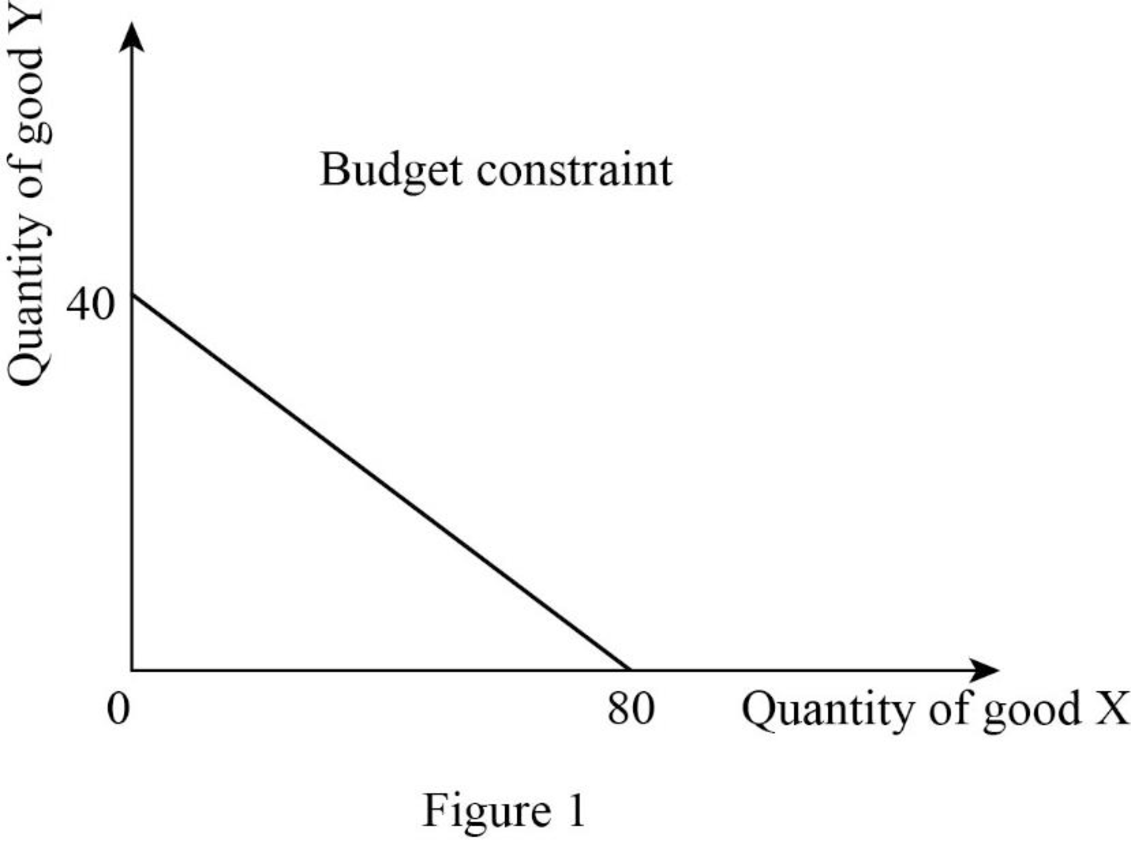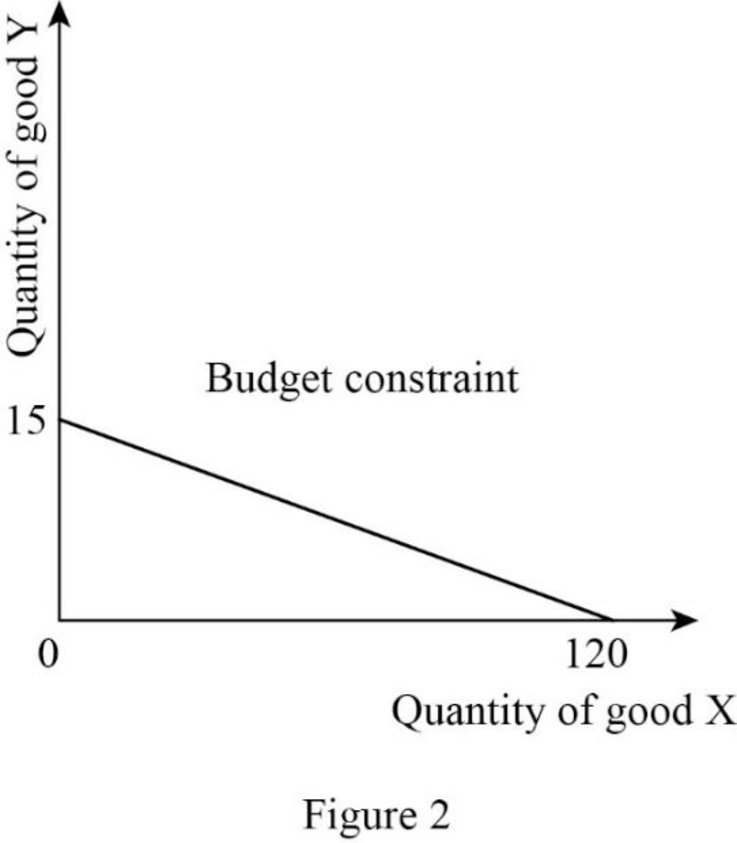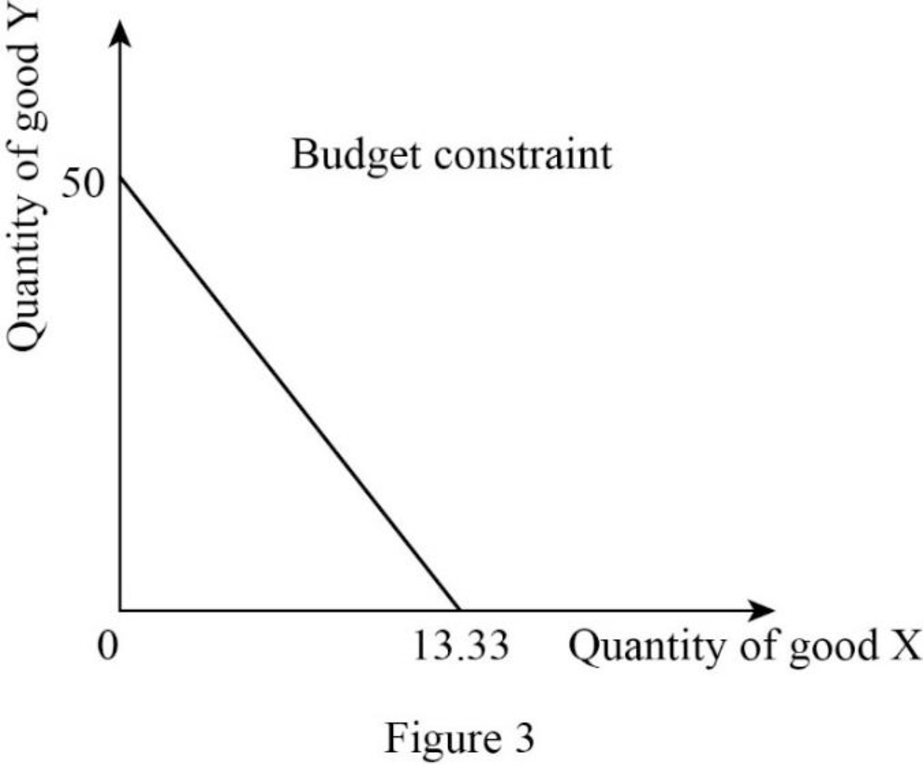Chapter E, Problem 1QP

Chapter
Section
Textbook Problem

Diagram the following budget constraints: a. Income = $4,000; PX =$50; PY = $100 b. Income =$3,000; PX = $25; PY =$200 c. Income = $2,000; PX =$40; PY = $150 (a) To determine Draw the budget constraint when income =$4,000; PX = $50; PY =$100.

Explanation

Since the income is $4,000 and price of good X is$50, the quantity of good X can be calculated as follows:

Quantity of good X=IncomePrice of good X=$4,000$50=80

Thus, the consumer will consume 80X.

Since the income is $4,000 and price of good Y is$100, the quantity of good Y can be calculated as follows:

Quantity of good Y=IncomePrice of good Y=$4,000$100=40

Thus, the consumer will consume 40Y.

Now, the budget constraint can be represented as follows:In Figure 1, the vertical axis measures the quantity of good Y and the horizontal axis measures the quantity of good X.

(b)

To determine

Draw the budget constraint when income = $3,000; PX =$25; PY = $200. Explanation Since the income is$3,000 and price of good X is $25, the quantity of good X can be calculated as follows: Quantity of good X=IncomePrice of good X=$3,000$25=120 Thus, the consumer will consume 120X. Since the income is$3,000 and price of good Y is $200, the quantity of good Y can be calculated as follows: Quantity of good Y=IncomePrice of good Y=$3,000$200=15 Thus, the consumer will consume 15Y. Now, the budget constraint can be represented as follows:In Figure 2, the vertical axis measures the quantity of good Y and the horizontal axis measures the quantity of good X. (c) To determine Draw the budget constraint when income =$2,000; PX = $40; PY =$150.

Explanation

Since the income is $2,000 and price of good X is$40, the quantity of good X can be calculated as follows:

Quantity of good X=IncomePrice of good X=$2,000$40=50

Thus, the consumer will consume 50X.

Since the income is $2,000 and price of good Y is$150, the quantity of good Y can be calculated as follows:

Quantity of good Y=IncomePrice of good Y=$2,000$150=13.33

Thus, the consumer will consume 13.33Y.

Now, the budget constraint can be represented as follows:In Figure 3, the vertical axis measures the quantity of good Y and the horizontal axis measures the quantity of good X.

The Solution to Your Study Problems

Bartleby provides explanations to thousands of textbook problems written by our experts, many with advanced degrees!

Get Started

Chapter E Solutions

What does the invisible hand of the marketplace do?

Principles of Microeconomics (MindTap Course List)

BOND YIELDS Last year Clark Company issued a 10-year, 12% semiannual coupon bond at its par value of 1,000. Cur...

Fundamentals of Financial Management, Concise Edition (with Thomson ONE - Business School Edition, 1 term (6 months) Printed Access Card) (MindTap Course List)

Explain how costs are assigned to activities.

Managerial Accounting: The Cornerstone of Business Decision-Making

Find all the answers to your study problems with bartleby.
Textbook solutions plus Q&A. Get As ASAP arrow_forward